• 给定正整数n，找到若干个完全平方数（比如1, 4, 9, 16, ...）使得它们的等于n。你需要让组成的完全平方数的个数最少。 示例1: 输入: n = 12 输出: 3 解释: 12 = 4 + 4 + 4. 示例 2: 输入: n = 13 输出: 2 ...

给定正整数 n，找到若干个完全平方数（比如 1, 4, 9, 16, ...）使得它们的和等于 n。你需要让组成和的完全平方数的个数最少。

示例 1:

输入: n =
12

输出: 3
解释:
12 = 4 + 4 + 4.
示例 2:

输入: n =
13

输出: 2
解释:
13 = 4 + 9.

很多人第一眼看到这个问题，想到的第一种做法就是使用贪心算法，但是对于这个问题是不适用的，例如：

如果贪心来做，12 = 9 + 1 + 1 + 1，所以答案为4了，但是实际答案是3。

Lagrange 四平方定理： 任何一个正整数都可以表示成不超过四个整数的平方之和。

引理 1 (Euler 四平方恒等式)： (a2+b2+c2+d2)(w2+x2+y2+z2) = (aw+bx+cy+dz)2 + (ax-bw-cz+dy)2 + (ay+bz-cw-dx)2 + (az-by+cx-dw)2， 其中 a, b, c, d, w, x, y, z 为任意整数。

引理 2： 如果一个偶数 2n 是两个平方数之和， 那么 n 也是两个平方数之和。

引理 3： 如果 p 是一个奇素数， 则存在正整数 k， 使得 kp = m2+n2+1 (其中 m， n 为整数)。

还有一个重要的推论：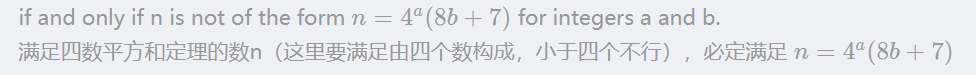我们可以先判断这个数是否满足，如果是，那就ans=4；

完整代码为

class Solution{
private:
int is_square(int n){
int sqrt_n = (int)(sqrt(n)); //强制转换 int i；float f； f=（float）i;
return (sqrt_n*sqrt_n == n);
}
public:
int numSquares(int n){
// If n is a perfect square, return 1.
if (is_square(n)){
return 1;
}
// The result is 4 if and only if n can be written in the
// form of 4^k*(8*m + 7). Please refer to
// Legendre's three-square theorem.
while ((n & 3) == 0) {// n%4 == 0
n >>= 2; //左移2位  4^a
}
if ((n & 7) == 7){ // n%8 == 7
return 4;
}
// Check whether 2 is the result.
int sqrt_n = (int)(sqrt(n));
for (int i = 1; i <= sqrt_n; i++){
if (is_square(n - i*i)){
return 2;
}
}
return 3;  //其他是3
}
};

不过这题用动态规划很容易理解

class Solution{
public:
int numSquares(int n)	{
if (n <= 0)		{
return 0;
}
vector<int> cntPerfectSquares(n + 1, INT_MAX);
cntPerfectSquares = 0;
for (int i = 1; i <= n; i++)	{
// For each i, it must be the sum of some number (i - j*j) and
// a perfect square number (j*j).
for (int j = 1; j*j <= i; j++)	{
cntPerfectSquares[i] = min(cntPerfectSquares[i], cntPerfectSquares[i - j*j] + 1);
}
}
return cntPerfectSquares.back();
}
};

展开全文c++
• 1．实验题目1.7【问题描述】第四平方和定理，又称为拉格朗日定理：每个正整数都可以表示为至多4个正整数的平方和。 如果把0包括进去，就正好可以表示为4个数的平方和。比如：5 = 0^2 + 0^2 + 1^2 + 2^2 7 = 1^2 + 1^...

1．实验题目

1.7【问题描述】

第四平方和定理，又称为拉格朗日定理：每个正整数都可以表示为至多4个正整数的平方和。  如果把0包括进去，就正好可以表示为4个数的平方和。比如：5 = 0^2 + 0^2 + 1^2 + 2^2 7 = 1^2 + 1^2 + 1^2 + 2^2(^符号表示乘方的意思)对于一个给定的正整数，可能存在多种平方和的表示法。 要求你对4个数排序：0 <= a <= b <= c <= d并对所有的可能表示法按a,b,c,d为联合主键升序排列，最后输出第一个表示法

【输入】  一个正整数N (N<5000000)

【输出】  4个非负整数，按从小到大排序，中间用空格分开

【输入范例1】 5

【输出范例1】0 0 1 2

【输入范例2】773535

【输出范例2】1 1 267 838

2．需求分析

本演示程序用VC++6.0编写，完成一个数经过拉格朗日定理所得结果。①输入的形式和输入值的范围：一个正整数N (N<5000000)。在所有输入中，元素的值都是整数②输出的形式：4个非负整数，按从小到大排序，中间用空格分开。③程序所能达到的功能：每个正整数都可以表示为至多4个正整数的平方和，找出其4个正整数

④测试数据：【输入范例1】 5

【输出范例1】0 0 1 2

【输入范例2】773535

【输出范例2】1 1 267 838

3．概要设计

1)为了实现上述程序功能，需要定义函数，主函数调用来实现：

int f(int n)

{

int a,b,c,d;

先定义四个形参；2)本程序包含2个函数：①主函数main()②求4个正整数函数int f(int n)各函数间关系如下：main()int f(int n)

4．详细设计

#include

#include

int MAX=(int)sqrt(5000000);          //利用最大值的开方数，使用sqrt函数

int f(int n)

{

int a,b,c,d;

for(a=0;a<=MAX;a++)          //5000000的开方数为MAX，a作为最外层的循环，即0<=a<=b<=c<=d，升序

{if(a*a>n)continue;      //满足a*a>n则结束此次循环，跳到下一语句

for(b=a;b<=MAX;b++)

{if(a*a+b*b>n)continue;     //满足a*a+b*b>n即结束此次循环

for(c=b;c<=MAX;c++)

{if(a*a+b*b+c*c>n)continue;  //满足a*a+b*b+c*c>n即结束此次循环

for(d=c;d<=MAX;d++)    //d变化最快

{if(a*a+b*b+c*c+d*d>n)continue;//满足a*a+b*b+c*c+d*d>n即结束此次循环

if(a*a+b*b+c*c+d*d!=n)continue;//判断4个正整数的平方和是否等于n,不等就结束此次循环

printf("%d %d %d %d\n",a,b,c,d);

return 0;               //输出满足条件的第一个表示法

}

}

}

}

return 0;

}

void main()

{

int n;

printf("请输入N值:");

scanf("%d/n",&n);         //输入一个整数

if(n<0||n>5000000)        //根据题目要求的范围

printf("输入的N值不在范围内!\n");

else f(n);

}

5．调试分析#include

#include

int MAX=(int)sqrt(5000000);

int f(int n)

{

int a,b,c,d;

for(a=0;a<=MAX;a++)

{if(a*a>n)continue;

for(b=a;b<=MAX;b++)

{if(a*a+b*b>n)continue;

for(c=b;c<=MAX;c++)

{if(a*a+b*b+c*c>n)continue;

for(d=c;d<=MAX;d++)

{if(a*a+b*b+c*c+d*d>n)continue;

if(a*a+b*b+c*c+d*d!=n)continue;

printf("%d %d %d %d\n",a,b,c,d);

return 0;

}

}

}

}

return 0;

}                                 //时间复杂度T(n)=O(n^4),空间复杂度S(n)=O(1)

void main()

{

int n;

printf("请输入N值:");

scanf("%d/n",&n);

if(n<0||n>5000000)

printf("输入的N值不在范围内!\n");

else f(n);

}

调试时，先进入main()函数，然后执行输出语句来进行提示，执行输入语句，再根据判断输入的n值来执行相应的语句，要是是1就输出“输入的N值不在范围内!”,要是0即调用f(n)函数。f(n)函数有四重循环，最外层为a的循环，接下来就是b,c,d。实现0 <= a <= b <= c <= d ，再根据判断四个数的平方和是否等于n，不等就结束此次循环，要是相等就输入结果，输出第一个就执行return 0语句，结束输出。

6．使用说明

程序名为1.exe，运行环境为VC++6.0。程序执行后显示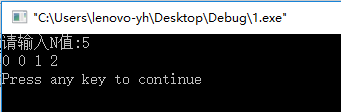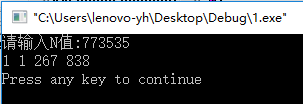根据提示，在第一行先输入一个正整数N (N<5000000)，点击换行，下一行输出结果。

7．测试结果

1)建立函数：

» 输入：5

输出：0 0 1 2

» 输入：773535

输出：1 1 267 838

» 输入：0

输出：输入的N值不在范围内!

展开全文• 目录 一，拉格朗日四平方和定理 二，证明过程 三，推论 ，OJ实战 CSU 1404 Four-square Theorem 力扣 279. 完全平方数 一，拉格朗日四平方和定理 每个正整数均可表示为4个整数的平方和 二，证明过程 1，欧拉恒等...

目录

一，拉格朗日四平方和定理

二，证明过程

三，推论

四，OJ实战

CSU 1404 Four-square Theorem

力扣 279. 完全平方数

一，拉格朗日四平方和定理

每个正整数均可表示为4个整数的平方和

二，证明过程

1，欧拉恒等式

2，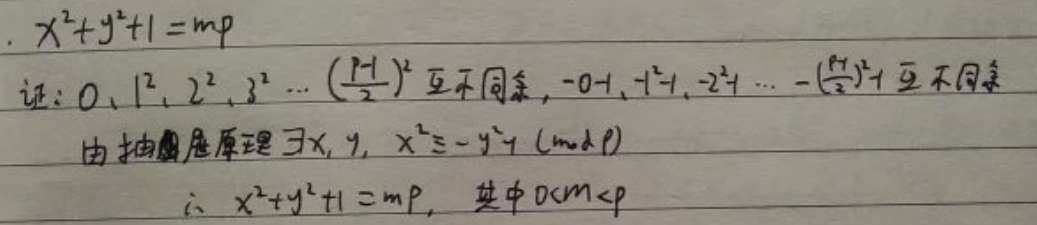3，任意素数都可以表示为四平方和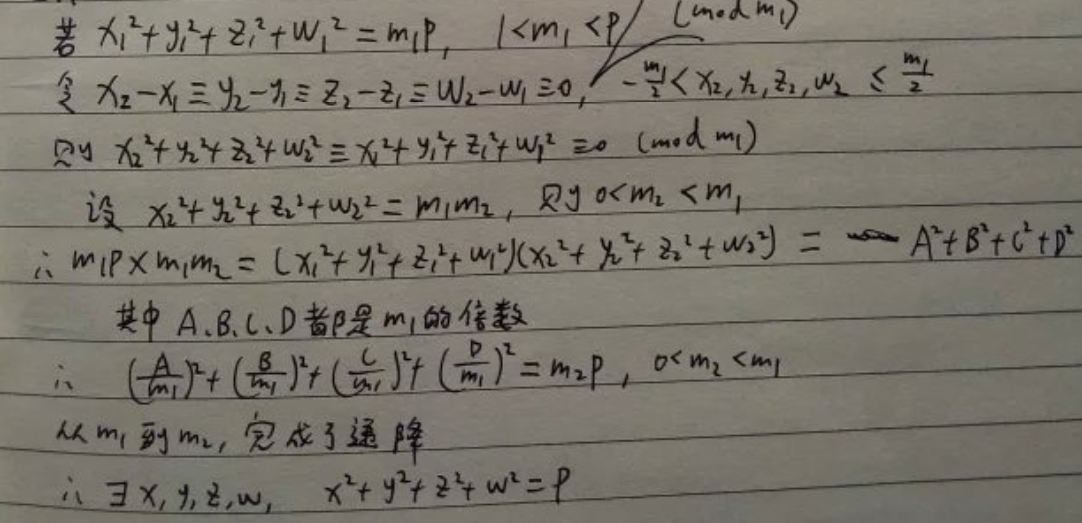三，推论

形如4^a * (8k+7)的数不能表示成三平方和，其他形式的数都可以表示成三平方和。

四，OJ实战

CSU 1404 Four-square Theorem

题目：

Description
Lagrange’s four-square theorem states that any natural number can be represented as the sum of four integer squares: n = a2 + b2 + c2 + d2. For example, 3, 31 and 310 can be represented as the sum of four squares as follows: 3 = 12 + 12 + 12 + 02, 31 = 52 + 22 + 12 + 12, 310 = 172 + 42 + 22 + 12.
Given an integer n, represent it by the sum of four integer squares.
This result may be helpful: Any integer n which is not of the form 4a(8m + 7) can be written as a sum of three squares, where a and m are arbitrary non-negative integers. For illustration, 7 = 40 * (8 * 0 + 7), so 7 can not be written as a sum of three squares.
Input
The first line contains the number of test cases T (1 <= T <= 104).
For each test case, there is only one line with an integer n (1 <= n <= 109).
Output
For each test case, output four integers a, b, c, d separated by a single space which satisfy n2 = a2 + b2 + c2 + d2. If there are multiple solutions, anyone will be accepted.

Sample Input
5
1
7
7
10
10
Sample Output
1 0 0 0
1 1 1 2
1 2 1 1
1 0 3 0
2 1 1 2

思路：

本来我的思路是利用欧拉四平方和恒等式，不过并没有什么效果，遇到8m+7型的大素数就直接变成暴力枚举了。

这个题目主要是根据给的提示，把不能表示成3个平方和的数拆分成一个尽量大的平方和加上一个可以表示成3个平方和的数

然后再暴力枚举

代码：

#include<iostream>
#include<math.h>
#include<stdio.h>
using namespace std;

bool g(int n)
{
while (n % 4 == 0)n /= 4;
return n % 8 == 7;
}

void f(int n)
{
for (int a = int(sqrt(n));; a--)
{
n -= a*a;
if (n && g(n))
{
n += a*a;
continue;
}
for (int b = 0; b*b <= n; b++)
{
n -= b*b;
for (int c = 0; c <= b && c*c <= n; c++)
{
n -= c*c;
int d = int(sqrt(n));
if (n == d*d)
{
printf("%d %d %d %d\n", a, b, c, d);
return;
}
n += c*c;
}
n += b*b;
}
}
}

int main()
{
int t, n;
scanf("%d", &t);
while (t--)
{
scanf("%d", &n);
f(n);
}
return 0;
}

力扣 279. 完全平方数

题目：

给定正整数 n，找到若干个完全平方数（比如 1, 4, 9, 16, ...）使得它们的和等于 n。你需要让组成和的完全平方数的个数最少。

示例 1:

输入: n = 12
输出: 3
解释: 12 = 4 + 4 + 4.
示例 2:

输入: n = 13
输出: 2
解释: 13 = 4 + 9.

思路一：

动态规划，枚举n的平方和组成中可能出现的值，只要枚举一个即可化为子问题。

代码：

class Solution {
public:
int numSquares(int n) {
static map<int,int>m;
if(m[n])return m[n];
m[n]=n;
for(int i=1;i*i<=n;i++)m[n]=min(m[n],numSquares(n-i*i)+1);
return m[n];
}
};

时间复杂度：O（n^(3/2)）

思路二：

用拉格朗日四平方和定理，所有的数都是四平方和。

判断一个数是不是平方数，如果不是的话，是不是平方和，如果不是的话，是不是三平方和，如果不是三平方和，那就是4。

先算出所有的平方，以及所有的平方和，然后用双指针扫描一遍看有没有加起来是n的。

时间复杂度O（n）

思路三：

用四平方和定理的推论，形如4^a * (8k+7)的数不能表示成三平方和，其他形式的数都可以表示成三平方和

只需要判断一个数是不是平方和即可。

用双指针的话，时间复杂度O（ sqrt（n））

暴力枚举平方和的话，O（n）

bool g(int n)
{
while (n % 4 == 0)n /= 4;
return n % 8 == 7;
}

class Solution {
public:
int numSquares(int n) {
if(g(n))return 4;
if(int(sqrt(n))*int(sqrt(n))==n)return 1;
for(int i=0;i<=100;i++)for(int j=0;j<=i;j++)if(n==i*i+j*j)return 2;
return 3;
}
};

展开全文算法 c++ 蓝桥杯
• 给定正整数n，找到若干个完全平方...拉格朗日四平方和定理： 1、对于任意一个正整数都可以表示成至多个整数的平方和(把0考虑进去了也) 下面剪枝性的来找对任意正整数n可以表示成多少几个整数的平方和 2、 若k%4=0...

给定正整数 n，找到若干个完全平方数（比如 1, 4, 9, 16, ...）使得它们的和等于 n。你需要让组成和的完全平方数的个数最少。
输入: n = 12 输出: 3 解释: 12 = 4 + 4 + 4.
拉格朗日四平方和定理：
1、对于任意一个正整数都可以表示成至多四个整数的平方和(把0考虑进去了也)
下面剪枝性的来找对任意正整数n可以表示成多少几个整数的平方和
2、 若k%4=0,则 k和n具有相同个数的整数和表示
3、 若n%8=7,则 n可以被表示为4个整数的平方和 n=4^a*(8b+7)

int init(){
while(n%4==0){
n/=4;
}
if(n%8==7) return 4;
for(int i=0;i*i<=n;++i){
int j=sqrt(n- i*i);
if(j*j == n-i*i){
return !!i + !!j;	//找1或 2的形式
}
}
return 3;
}

看讨论区有个用bfs来搜，从n搜到0，看完后敲了dfs,对于有些数复杂度爆炸

int dfs(int n){
if(!n)return 0;
if(sqrt(n)*sqrt(n)==n){
return 1;
}
int cnt=0x3f3f3f;
for(int i=1;i*i<=n;++i){
cnt=min(cnt,dfs(n-i*i)+1);
}
return cnt;
}

bfs可以这样理解：给出一个正整数n找到若干个完全平平方数使得n减去他们的差为0，求最少的完全平方数

#include <bits/stdc++.h>
using namespace std;
const int maxn=1e6;
const int inf=0x3f3f3f3f;
int dp[maxn];
int a[maxn];
int n;
int cnt;
void  bfs(){
queue< pair<int,int> >Q;
Q.push(make_pair(n,0) );
vector<bool> vis(n,false);
vis[n]=true;
while(!Q.empty()){
int num= Q.front().first;
int step= Q.front().second;
Q.pop();
for(int i=1;i*i<=num;++i){
int a=num - i*i;
if(a<0) break;
else if( a== 0) {
cnt =step+1;
return ;
}
else{
if(!vis[a]){
Q.push(make_pair(a,step+1));
vis[a]=true;
}
}
}
}
}
int main(){
while(scanf("%d",&n)!=EOF){
bfs();
cout<<cnt<<endl;
}
return 0;
}

递推性的dp

memset(dp,maxn,sizeof(dp));
dp=0;
for(int i=1;i<=n;++i){
for(int j=1;j*j<=i;++j){
dp[i]=min(dp[i],dp[i-j*j]+1);
}
}
cout<<dp[n]<<endl;

展开全文• 四平方和(C++)

2021-03-31 08:41:40
四平方和定理，又称为拉格朗日定理： 每个正整数都可以表示为至多4个正整数的平方和。 如果把0包括进去，就正好可以表示为4个数的平方和。 比如： 5 = 02 + 02 + 12 + 22 7 = 12 + 12 + 12 + 22 对于一个给定的正...c++
• 问题描述：四平方和定理，又称为拉格朗日定理：每个正整数都可以表示为至多个正整数的平方和。如果把 00 包括进去，就正好可以表示为个数的平方和。比如：5=02+02+12+227=12+12+12+22则对于一个给定的正整数 ...
• 1. 四平方和定理 定理表明 每个正整数均可表示为4个整数的平方和。也就是说这个题的答案，我们可以先在数组中预处理出平方和，然后两层for循环暴力循环判断1，2，顺便可以由剩下的检验一下是不是3，都不是的话，...leetcode dp 四平方和定理 背包 c++
• 拉格朗日四平方和定理： 每一个非负整数都可以表示成个非负整数的平方和。 例如 5 = 0^2 + 0^2 + 1^2 + 2^2 给定一个正整数n，请你将n拆成 a^2+b^2+c^2+d^2，问 a+b+c+d最小是多少。 输入格式： 一个正整数...算法 动态规划
• 拉格朗日四平方和定理：任意一个非负整数都能表示成个平方数的和（这里0也算是平方数） 注意这个题里0不算是平方数。 然后169这个数字有个奇特的性质，就是它可以分别用1、2、3、4、5个平方数的和表示出来，这样...c++
• #include<stdio.h> int main(void){ int a,b,c,d; int number; scanf("%d",&number);...c++) { for(d=0;d<1000;d++) { if(aa+bb+cc+dd==number) { if(d>=c&
• 如果把0包括进去，就正好可以表示为4个数的平方和。 比如： 5 = 0^2 + 0^2 + 1^2 + 2^2 7 = 1^2 + 1^2 + 1^2 + 2^2 （^符号表示乘方的意思） 对于一个给定的正整数，可能存在多种平方和的表示法。 要求你对4个数排序...蓝桥杯 四平方和定理
• #include <stdio.h> #include <math.h> int main() {  int a, b, c, n, flag = 0;  double maxN, d; printf("输入一个正整数为：");  scanf("%d", &n);...dev-c++编写。   6. 测试结果
• c++) for(int d=c; d; d++) if(a*a+b*b+c*c+d*d==n) { printf("%d %d %d %d",a,b,c,d); return 0; } return 0; } 2018年03月29日17:10:02更新，可以采取更巧妙一点的方法 #include #include int mpt...
• 记录一下四平方和定理的解法 int numSquares(int n) { while (0 == (n & 0x3)) { n >>= 2; } if (7 == (n & 0x7)) { return 4; } for (int i = sqrt(n); i > 0; --i) { int j = ...c++ 四平方定理
• 【C++】四方定理

千次阅读 2016-01-05 11:36:00
四方定理的内容是：所有的自然数至多只要用4个数的平方和就可以表示。编程验证该定理 #include"iostream.h" #include"stdio.h" //四方定理的内容是：所有的自然数至多只要用4个数的平方和就可以表示。编程验证该...
• 蓝桥杯b组c++.8 四平方定理

千次阅读 2016-04-07 09:50:30
平方和四平方和定理，又称为拉格朗日定理： 每个正整数都可以表示为至多4个正整数的平方和。 如果把0包括进去，就正好可以表示为4个数的平方和。比如： 5 = 0^2 + 0^2 + 1^2 + 2^2 7 = 1^2 + 1^2 + 1^2 + 2^2 ...c语言
• 2016年第七届蓝桥杯题目汇总 ... 四平方和定理，又称为拉格朗日定理： 每个正整数都可以表示为至多4个正整数的平方和。 如果把0包括进去，就正好可以表示为4个数的平方和。 比如： 5 = 0^2 +...C++ 蓝桥杯
• #include using namespace std; int main() { int number,i,j,k,l; cout输入一个数"; cin>>number; for(i=1;i;i++) for(j=0;j;j++) for(k=0;k;k++) for(l=0;l;l++) if(numb
• 四平方和定理，又称为拉格朗日定理： 每个正整数都可以表示为至多4个正整数的平方和。 如果把0包括进去，就正好可以表示为4个数的平方和。 比如： 5 = 0^2 + 0^2 + 1^2 + 2^2 7 = 1^2 + 1^2 + 1^2 + 2^2 （^符号表示...蓝桥杯 c++
• 更多精彩文章请关注公众号『大海的BLOG』问题：四平方和定理，又称为拉格朗日定理：每个正整数都可以表示为至多4个正整数的平方和。如果把0包括进去，就正好可以表示为4个数的平方和。比如：5 = 0^2 + 0^2 + 1^2 + 2...
• 四平方和定理，又称为拉格朗日定理： 每个正整数都可以表示为至多4个正整数的平方和。 如果把0包括进去，就正好可以表示为4个数的平方和。 比如： 5 = 0^ 2 + 0^ 2 + 1^ 2 + 2^2 7 = 1^ 2 + 1^ 2 + 1^ 2 + 2^2 （^...
• //第七届蓝桥杯软件类省赛真题-C-B-8_四平方和定理 /* 四平方和定理，又称为拉格朗日定理： 每个正整数都可以表示为至多4个正整数的平方和。 如果把0包括进去，就正好可以表示为4个数的平方和。 比如： 5 = 0^2...
• 此题直接循环，但没必要四重循环 #include #include int main() { int n,a,b,c,d; scanf("%d",&n); for(a=0;a;a++)//N { for(b=a;b;b++) { for(c=b;c;c++)//当蓝桥杯
• C++四平方和

千次阅读 2018-03-24 20:21:44
四平方和定理，又称为拉格朗日定理：每个正整数都可以表示为至多4个正整数的平方和。如果把0包括进去，就正好可以表示为4个数的平方和。比如：5 = 0^2 + 0^2 + 1^2 + 2^27 = 1^2 + 1^2 + 1^2 + 2^2（^符号表示乘方的...
• 第七届蓝桥杯省赛C++B组 四平方和

千次阅读 热门讨论 2018-03-22 13:17:26
四平方和定理，又称为拉格朗日定理： 每个正整数都可以表示为至多4个正整数的平方和。 如果把0包括进去，就正好可以表示为4个数的平方和。 比如： 5 = 0^2 + 0^2 + 1^2 + 2^2 7 = 1^2 + 1^2 + 1^2 + 2^2 （^符号表示...递归
• 数论中有著名的四方定理：所有自然数至多只要用个数的平方和就可以表示。 我们可以通过计算机验证其在有限范围的正确性。 对于大数，简单的循环嵌套是不适宜的。下面的代码给出了一种分解方案。 请仔细阅读，...
• java实现第七届蓝桥杯四平方和

万次阅读 多人点赞 2019-07-29 12:19:26
四平方和定理，又称为拉格朗日定理： 每个正整数都可以表示为至多4个正整数的平方和。 如果把0包括进去，就正好可以表示为4个数的平方和。 比如： 5 = 0^2 + 0^2 + 1^2 + 2^2 7 = 1^2 + 1^2 + 1^2 + 2^2 （^符号表示...java 第七届蓝桥杯 四平方和
• 力扣 LeetCode 完全平方C++实现 题目 给定正整数 n，找到若干个完全平方数（比如 1, 4, 9, 16, …）使得它们的和等于 n。你需要让组成和的完全平方数的个数...此前不知道有一个“四平方和定理”，如果应用该定...c++ 算法 leetcode...

四平方和定理c++c++ 订阅